# Understanding Point Processes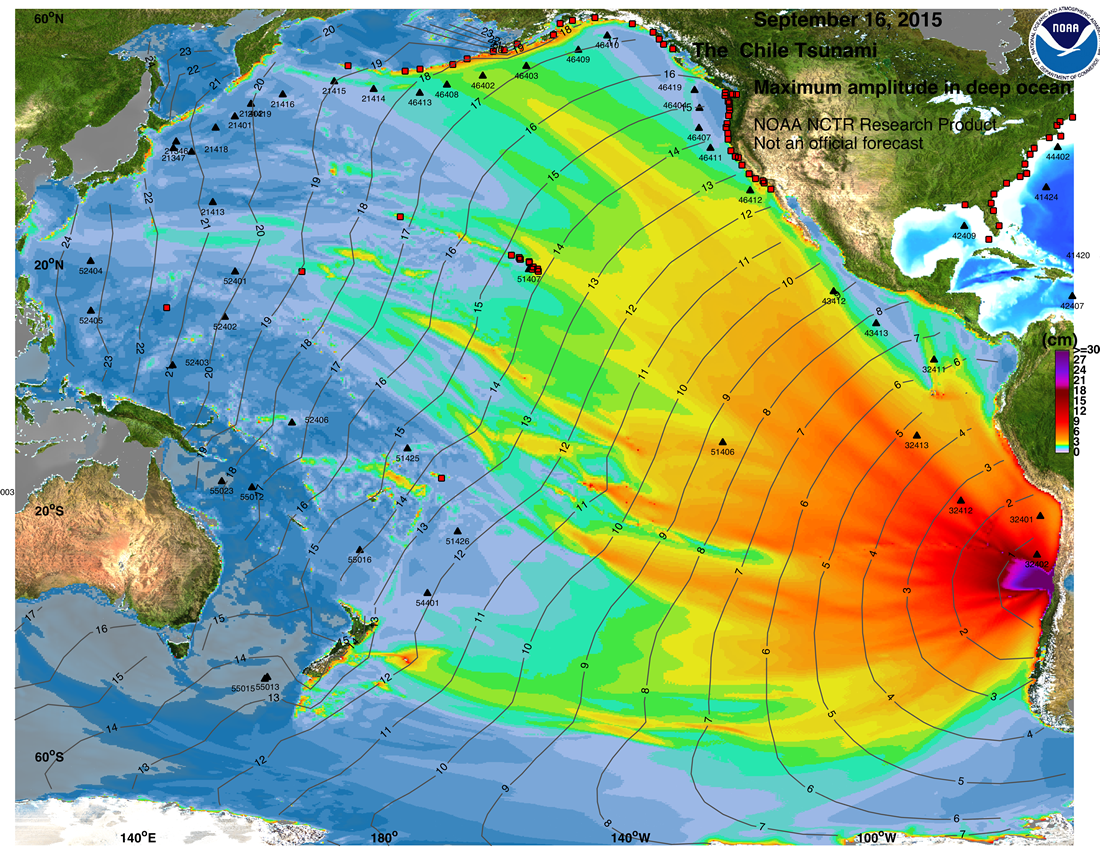Maximum tsunami wave height generated by the 16 Sept. 2015 Chile earthquake, from the International Tsunami Information Center. Posted by Austin Elliott

In this world, many events occur and their trends are likely to follow a pattern. In this blog, we try to lay foundations to model those patterns. For example, the likeliness of a new earthquake typically increases in the region where an earthquake has already occurred. This increase in likeliness can be mainly because of the aftershocks created by the earlier earthquake. A panic selling of stocks in one country can cause a similar event in a different country.

Take an example of wildfire, a wildfire in an amazon forest this year can greatly decrease the occurrence of another wildfire in the coming year. This decrease in the likeliness of wildfire next year is mainly because of the combustion of existing forest fuel. So it is clear that probabilities of a similar event can be elevated or decreased — by patterns in the sequence of previous events.

If the probabilities of a similar event are elevated i.e., each occurrence increases the rate of future occurrence, like in earthquakes example, then these events can be categorised as stochastically excited or self- excited. If the probability of a similar event is decreased, like in earthquakes example then these events can be categorised as stochastically inhibited or self-regulating. If the probability of a similar event is unaffected, each occurrence doesn’t have any impact on the rate of future occurrence, then these events can modelled as a Poisson point process

# Theory of Point Process

A point process is a stochastic model underlying the occurrence of events in time and/or space. In this blog, we will emphasis on purely temporal aspects of point process i.e., the space in which the points fall is simply a portion of the real line which represents time.

To start, consider a line that represents time and event times T₁,T₂,… of event times falling along the line, T (event times ) can usually be interpreted as the time of occurrence of the i-th event. This event can be earthquake in a particular region or wildfire in amazon forest. Our job is to model these event times. Instead of modelling these event times T₁,T₂ ,… Tn, it can alternatively be described by a counting process N(t).

A counting process N(t) can be viewed as a cumulative count of the number of ‘arrivals’ into a system up to the current time t. If 146 earthquakes had occurred in Himalayans for last 80 years since the seismograph in installed, the N(80) = 146. Simple enough right!
let’s also define history: H(u) history of the arrivals up to time u.

When we are discussing the concepts of stochasticity, it is pertinent to define a function that gives the expectation of event occurrence at time t. That function is called intensity function and denotes as λ٭(t), which represents the infinitesimal rate at which events are expected to occur around a particular time t. It is conditional on the prior history H(t) of the point process prior to time t.

The behaviour of a simple temporal point process N(t) is typically modeled by specifying its conditional intensity, λ٭(t).

We introduced terms like ‘self-exciting’ and ‘self-regulating’ which can be easily understood using the conditional intensity function. If a recent arrival in history H(t) causes the conditional intensity function to increase then the process is said to be self-exciting. In general, λ٭(t) depends not only on t but also on the times T of preceding events i.e., is H(t).
When N is Poisson point process, the conditional intensity function λ٭(t) depends only on information about the current time, but not on history H(u). Poisson point process is neither self-exciting nor self-regulating.
λ٭(t) is just function of over time for Poisson point process, stationary Poisson process has constant conditional rate: λ٭(t) = α, for all t. λ٭(t) = α implies that the probability of occurrence of an event is constant at any point of time regardless of how frequently such events have occurred previously.

# Hawkes process

The Hawkes process belongs to a family of self-exciting point processes named after its creator Alan G. Hawkes. Self-exciting point process models are used model events that are temporally clustered. Events like “earthquakes” and “panic selling of stocks” are often temporally clustered, i.e., the arrival of an event increases the likelihood of observing such events in the near future. Let’s define the Hawkes conditional intensity function —
Definition {t1, t2, . . . , tk} to denote the observed sequence of past arrival times of the point process up to time t, the Hawkes conditional intensity is

The constant λ is called background intensity, μ(·) is called excitation function. if μ(·) equals zero then this self-exciting point process reduces to simple stationary poisson process. A common choice for excitation function, μ(·) is exponential decay.

Parameters α and β are the constants. α, β can be interpreted as that each arrival in the system instantaneously increases the arrival intensity by α, then over time, this arrival’s influence decays at rate β.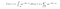Hawkes Conditional Intensity Function

The modified Hawkes conditional intensity looks like this. Another frequently used excitation function in power law function.

α and β are the parameters of λ٭(t) and let θ represent parameters. The parameter vector θ for a point process is estimated by maximizing the log-likelihood function. We can also use parametric functions to approximate conditional intensity function, we will discuss more about that in the next blog in this blog series.
There is an obvious extension to the self exciting point process which is mutually-exciting point process. These are essentially set of one-dimensional point processes which excite themselves and each other. This set of point process are called multi-variate or mutually exciting point processes.

If for each i = 1, . . . , m then each counting process N(t) has conditional intensity of the form:mutually exciting Hawkes process

# Simulations

Let’s simulate a simple Hawkes point process: λ: 0.1, α:0.1, β:0.1 and try to understand conditional intensity function.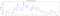Figure 1: Conditional Intensity Function

We can clearly observe the excitation and decay in the above graph. Now let’s increase the background intensity λ to 0.5.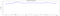Figure 2

We clearly see the number of events increased as the background intensity increased and hovered above 0.5. We will now try to understand the impact of α, β. Let’s increase α to 0.5.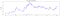Figure 3

We can clearly see that the number of events increased, this is because each occurrence of event increases the arrival intensity of next event by α. So λ٭(t) increase became higher, also one interesting observation is that λ٭(t) varied from 0.1 to 0.6.

Now let's increase the β to 0.5. Remember β controls the influence of decay rate of an event on its successive event.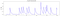Figure 4

Compare this plot with Fig 1, the decay is in Fig 1 is very less than the decay in Fig 4.
The core concepts of Hawkes point process are demonstrated in the above examples.

Point processes have extensive applications in various fields. We can model streams of discrete event/events in continuous time. We can also use function approximations where the conditional intensity functions of multiple event type can be approximated by novel neural architectures like LSTM. In the next blog in this series, we will discuss neural hawkes process.

Thanks for your time😇

RL | ML | ALGO TRADING | TRANSPORTATION | GAME THEORY

RL | ML | ALGO TRADING | TRANSPORTATION | GAME THEORY